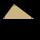# Right triangle + expression of a variable from the formula - math problems

#### Number of problems found: 329

• Isosceles right triangleArea of an isosceles right triangle is 18 dm2. Calculate the length of its base.
• Right-angled triangleDetermine the content of a right triangle whose side lengths form successive members of an arithmetic progression and the radius of the circle described by the triangle is 5 cm.
• Right triangle eq2Find the lengths of the sides and the angles in the right triangle. Given area S = 210 and perimeter o = 70.
• Right triangle - ratioThe lengths of the legs of the right triangle ABC are in ratio b = 2: 3. The hypotenuse is 10 cm long. Calculate the lengths of the legs of that triangle.
• Right triangleA circle with a radius of 5 cm is described in a right triangle with a 6 cm leg. What is the height at the hypotenuse of this triangle?
• Right triangleLegs of the right triangle are in the ratio a:b = 2:8. The hypotenuse has a length of 87 cm. Calculate the perimeter and area of the triangle.
• Integer sidesA right triangle with an integer length of two sides has one leg √11 long. How much is its longest side?
• Right angleIn a right triangle ABC with a right angle at the apex C, we know the side length AB = 24 cm and the angle at the vertex B = 71°. Calculate the length of the legs of the triangle.
• The right triangleThe right triangle ABC has a leg a = 36 cm and an area S = 540 cm2. Calculate the length of the leg b and the median t2 to side b.
• Right isoscelesCalculate area of the isosceles right triangle which perimeter is 41 cm.
• CalculateCalculate the length of a side of the equilateral triangle with an area of 50cm2.
• Right ΔA right triangle has the length of one leg 11 cm and the hypotenuse 61 cm size. Calculate the height of the triangle.
• Angle in RTDetermine the size of the smallest internal angle of a right triangle whose sides constitutes sizes consecutive members of arithmetic progressions.
• Equilateral triangleCalculate the area of an equilateral triangle with circumference 72cm.
• Equilateral triangleThe equilateral triangle has a 23 cm long side. Calculate its content area.
• Right triangleIt is given a right triangle angle alpha of 90 degrees beta angle of 55 degrees c = 10 cm use Pythagorean theorem to calculate sides a and b
• Difference of legsIn a right triangle, the length of the hypotenuse is 65 m, and the difference of legs is 23 m. Calculate the perimeter of this triangle.
• Medians in right triangleIt is given a right triangle, angle C is 90 degrees. I know it medians t1 = 8 cm and median t2 = 12 cm. .. How to calculate the length of the sides?
• Sides of right angled triangleOne leg is 1 m shorter than the hypotenuse, and the second leg is 2 m shorter than the hypotenuse. Find the lengths of all sides of the right-angled triangle.
• Right angled triangleHypotenuse of a right triangle is 17 cm long. When we decrease length of legs by 3 cm then decrease its hypotenuse by 4 cm. Determine the size of legs.

Do you have an interesting mathematical word problem that you can't solve it? Submit a math problem, and we can try to solve it.

We will send a solution to your e-mail address. Solved examples are also published here. Please enter the e-mail correctly and check whether you don't have a full mailbox.

Please do not submit problems from current active competitions such as Mathematical Olympiad, correspondence seminars etc...